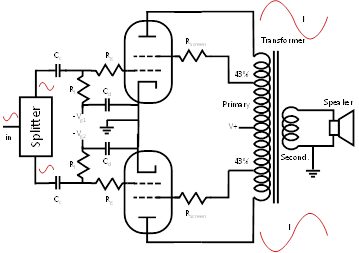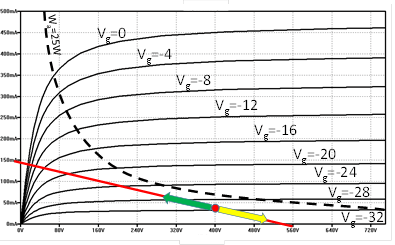In a push-pull vacuum tube amplifier, two power vacuum tubes are used to amplify phase-inverted copies of the same signal. The phase splitter stage (discussed later in Section 4.2), before the push-pull power stage, creates the two phase-inverted copies of the same input signal. The two phase-inverted signals, amplified by the two power tubes, are combined together using an output transformer appositively conceived for push-pull configuration.

The basic schema of a push-pull amplifier stage is depicted in Figure 20. It is composed of two vacuum tubes having an identical configuration and circuitry. The primary of the push-pull output transformer has a centre tap that receives the V+ voltage. Anodes of the two vacuum tubes are connected to the two ends of the output transformer primary. Similarly, the two taps for ultra-linear configuration are connected to the two screens of the vacuum tubes. If we consider just half transformer and one vacuum tube, the schema is practically identical to the single ended configuration. A push-pull stage can be seen as two single ended stages connected together through the push-pull output transformer. When no signal is applied to phase splitter, the two power vacuum tubes receive no signal and just the bias current goes from the anodes to the cathodes. The current enters from the transformer primary centre tap and flows in opposite direction through the transformer toward the first and the second vacuum tube.Figure 20: Basic schema of a push-pull amplifier.The phase splitter, which will be discussed later, takes the input signal and returns two copies of the same signal each with phase inverted with respect to the other. The two signals are given to two vacuum tubes that amplify them separately. The primary of the output transformer, used for a push-pull stage, has a centre tap that takes the high voltage. The two ends of the primary go to the anodes of the two vacuum tubes. Given that the two vacuum tubes amplify signals that are 180° one from the other, in the two ends of the transformer current varies symmetrically. That is, when in one end current increases, in the other end current decreases.

When a signal is received by the two vacuum tubes, the two amplified signals are identical, with inverted phases, and make the current to vary symmetrically, with respect to the bias current, in the two halves of the push-pull transformer. When in one half of the transformer current increases, with respect to the bias current, in the other half current decreases. The same effect happens to the voltage measured at the two anodes of the two vacuum tubes. On the load line, this effect is seen as movements in opposite directions, as depicted in Figure 21. While one tube moves following the green arrow, the other one moves following the yellow arrow, and vice versa.

The power vacuum tubes used in a push-pull power stage should be perfectly matched to guarantee a symmetrical behaviour. In order to fine tune the two power vacuum tubes, the two bias voltages -Vg1 and -Vg2 should be adjustable so that the quiescent bias current is exactly the same in the two tubes.Figure 21: Dynamic behaviour of a push-pull amplifier.When quiescent, the two power vacuum tubes are at the same operating point, depicted by the red spot. When a signal is amplified, since the vacuum tubes receive signals with inverted phases, they move in opposite directions. When one conducts more, as depicted by the green arrow, the other conducts less, as shown by the yellow arrow.